# 10 個適用於初學者和高級用戶的 VLOOKUP 示例

VLOOKUP 函數是基準。

### 何時在 Excel 中使用 VLOOKUP 函數？

VLOOKUP 函數最適合您在列中查找匹配數據點的情況，當找到匹配數據點時，您會在該行的右側並從指定數量的單元格中獲取值右側的列。

• 您走到佈告欄並開始尋找您的姓名或註冊號（在列表中從上到下移動您的手指）。
• 一旦你發現你的名字，你就會把眼睛移到名字/註冊號的右邊來查看你的分數。

VLOOKUP 函數在列中查找指定值（在上面的示例中，它是您的名字），當它找到指定的匹配項時，它會在同一行中返回一個值（您獲得的標記）。

### 句法

=VLOOKUP(lookup_value, table_array, col_index_num, [range_lookup])

### 輸入參數

• lookup_value –這是您試圖在表的最左列中查找的查找值。它可以是一個值、一個單元格引用或一個文本字符串。在記分錶示例中，這將是您的姓名。
• table_array –這是您要在其中查找值的表數組。這可以是對單元格範圍或命名範圍的引用。在分數表示例中，這將是包含每個主題的每個人的分數的整個表
• col_index –這是您要從中獲取匹配值的列索引號。在分數表示例中，如果您想要數學的分數（這是包含分數的表中的第一列），您可以查看第 1 列。如果您想要物理的分數，您可以查看第 1 列2.
• [range_lookup] –在這裡您可以指定您想要精確匹配還是近似匹配。如果省略，則默認為 TRUE – 近似匹配（請參閱下面的附加說明）。

### 附加說明（無聊，但重要的是要知道）

• 匹配可以是精確的（FALSE 或 range_lookup 中的 0）或近似的（TRUE 或 1）。
• 在近似查找中，確保列表按升序（從上到下）排序，否則結果可能不准確。
• 當 range_lookup 為 TRUE（近似查找）並且數據按升序排序時：
• 如果 VLOOKUP 函數找不到該值，則返回小於 lookup_value 的最大值。
• 如果lookup_value 小於最小值，則返回#N/A 錯誤。
• 如果lookup_value 是文本，可以使用通配符（參考下面的示例）。

## 10 個 Excel VLOOKUP 示例（基礎和高級）

### 示例 1 – 查找 Brad 的數學分數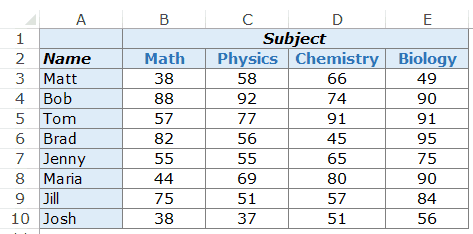`=VLOOKUP("Brad",\$A\$3:\$E\$10,2,0)`

• \$A\$3:\$E\$10 – 這是我們正在查看的單元格範圍。請記住，Excel 在最左側的列中查找查找值。在此示例中，它將在 A3:A10 （這是指定數組的最左側列）中查找名稱 Brad。
• 0 – 這告訴 VLOOKUP 函數只查找完全匹配。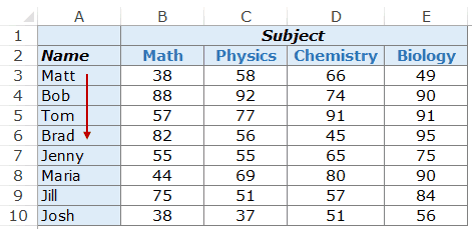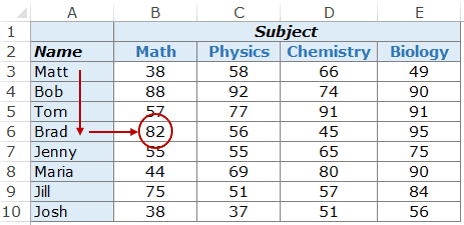`=VLOOKUP("Maria",\$A\$3:\$E\$10,4,0)`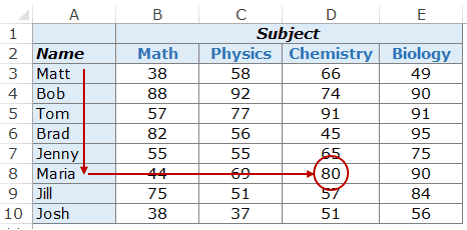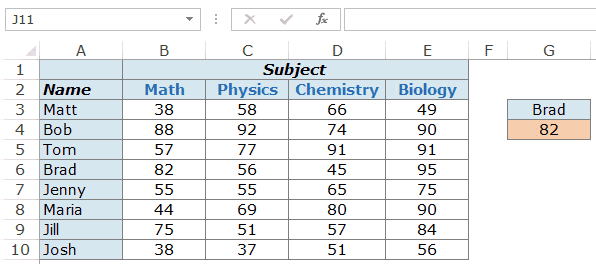### 示例 2 – 雙向查找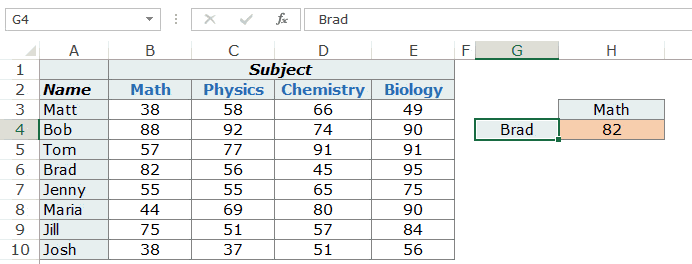`=VLOOKUP(G4,\$A\$3:\$E\$10,MATCH(H3,\$A\$2:\$E\$2,0),0)`

### 示例 3 – 使用下拉列表作為查找值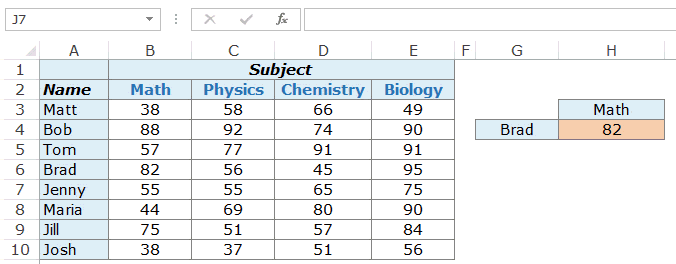`=VLOOKUP(G4,\$A\$3:\$E\$10,MATCH(H3,\$A\$2:\$E\$2,0),0)`

• 選擇需要下拉列表的單元格。在此示例中，在 G4 中，我們需要學生姓名。
• 轉到數據 -> 數據工具 -> 數據驗證。
• 在數據驗證對話框的設置選項卡中，從允許下拉列表中選擇列表。
• 在源中，選擇 \$A\$3:\$A\$10
• 單擊確定。

### 示例 4 – 三向查找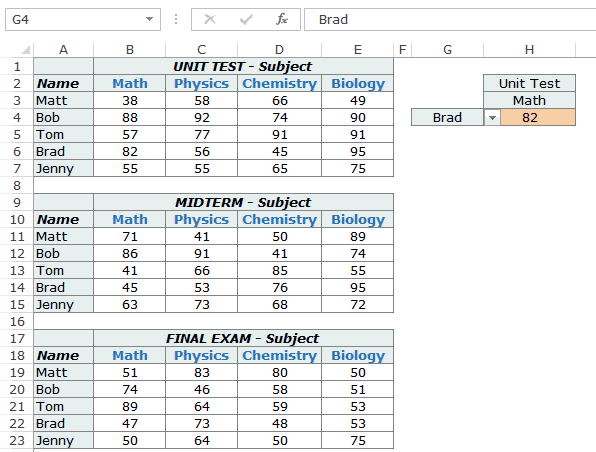`=VLOOKUP(G4,CHOOSE(IF(H2="Unit Test",1,IF(H2="Midterm",2,3)),\$A\$3:\$E\$7,\$A\$11:\$E\$15,\$A\$19:\$E\$23),MATCH(H3,\$A\$2:\$E\$2,0),0)`

CHOOSE(IF(H2=”Unit Test”,1,IF(H2=”Midterm”,2,3)),\$A\$3:\$E\$7,\$A\$11:\$E\$15,\$A\$19:\$E\$23)

### 示例 5 – 從列表中獲取最後一個值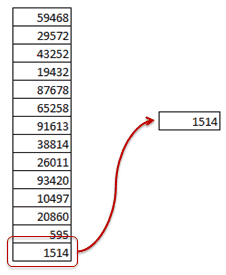`=VLOOKUP(9.99999999999999E+307,\$A\$1:\$A\$14,TRUE)`

• 如果找到完全匹配，則返回該值。
• 如果它找到一個高於查找值的值，則返回其上方單元格中的值。
• 如果查找值大於列表中的所有值，則返回最後一個值。

`=VLOOKUP("zzz",\$A\$1:\$A\$8,1, TRUE )`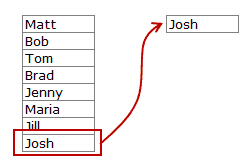### 示例 6 – 使用通配符和 VLOOKUP 進行部分查找

Excel 通配符在許多情況下都非常有用。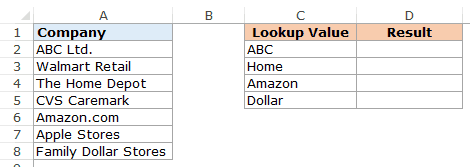`=VLOOKUP("*"&C2&"*",\$A\$2:\$A\$8,1,FALSE)`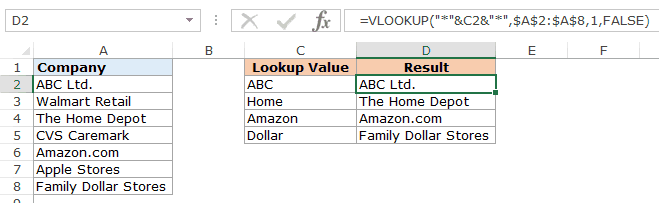### 示例 7 – 儘管查找值匹配，但 VLOOKUP 返回錯誤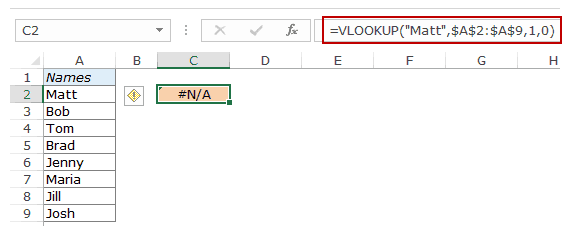`=VLOOKUP("Matt",TRIM(\$A\$2:\$A\$9),1,0)`

### 示例 8 – 進行區分大小寫的查找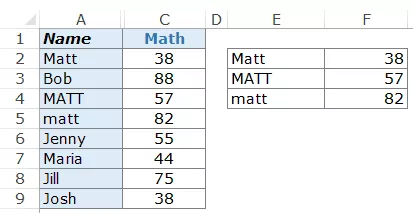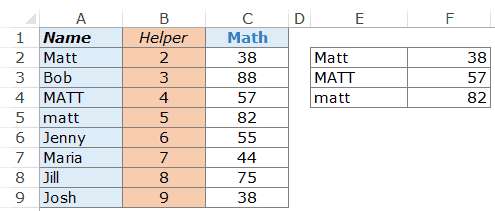`=VLOOKUP(MAX(EXACT(E2,\$A\$2:\$A\$9)*(ROW(\$A\$2:\$A\$9))),\$B\$2:\$C\$9,2,0)`

• EXACT(E2,\$A\$2:\$A\$9) – 這部分會將 E2 中的查找值與 A2:A9 中的所有值進行比較。它返回一個 TRUE/FALSE 數組，其中 TRUE 在完全匹配的情況下返回。在這種情況下，它將返回以下數組：{TRUE;FALSE;FALSE;FALSE;FALSE;FALSE;FALSE;FALSE}。
• EXACT(E2,\$A\$2:\$A\$9)*(ROW(\$A\$2:\$A\$9) – 這部分將 TRUE/FALSE 數組與行號相乘。只要有 TRUE，它就會給出行號，否則它給出 0。在這種情況下，它將返回 {2;0;0;0;0;0;0;0}。
• MAX(EXACT(E2,\$A\$2:\$A\$9)*(ROW(\$A\$2:\$A\$9))) – 這部分返回數字數組中的最大值。在這種情況下，它將返回 2（這是完全匹配的行號）。
• 現在我們只需將此數字用作查找值並將查找數組用作 B2:C9

### 示例 9 – 將 VLOOKUP 與多個條件一起使用

Excel VLOOKUP 函數，在其基本形式中，可以查找一個查找值並從指定的行返回相應的值。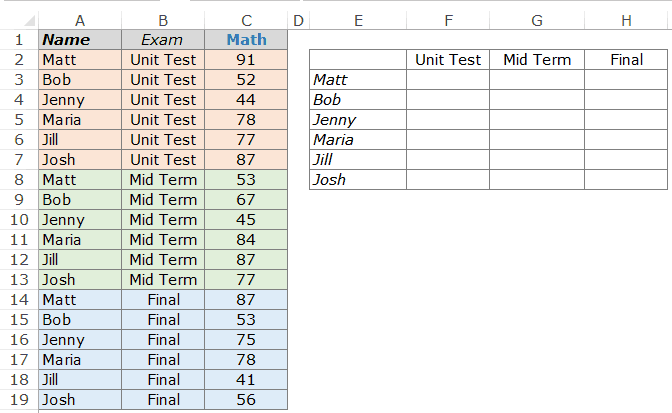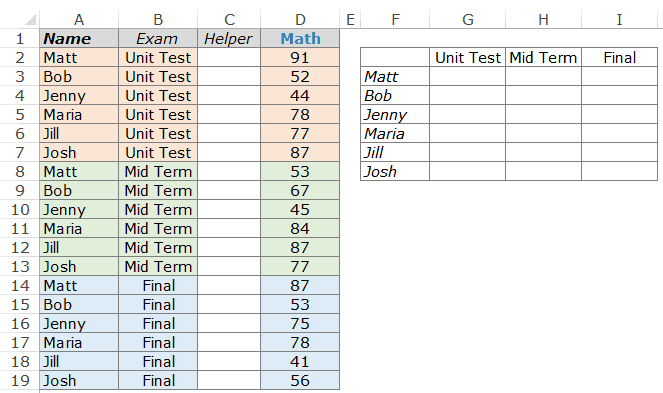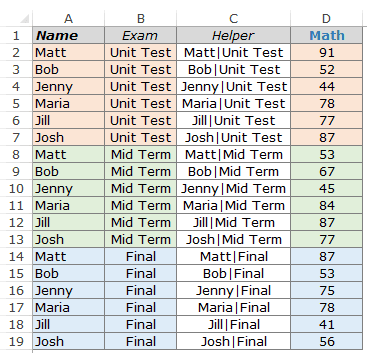`=VLOOKUP(\$F3&"|"&G\$2,\$C\$2:\$D\$19,2,0)`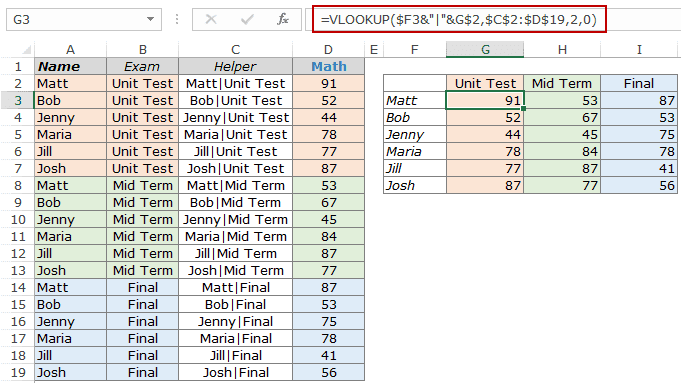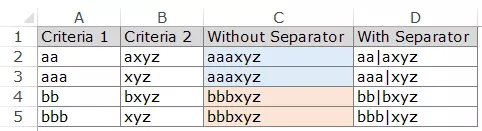### 示例 10 – 在使用 VLOOKUP 函數時處理錯誤

Excel VLOOKUP 函數在找不到指定的查找值時返回錯誤。如果 VLOOKUP 找不到值，您可能不希望醜陋的錯誤值干擾數據的美觀。`=IFERROR(VLOOKUP(D2,\$A\$2:\$B\$7,2,0),"Not Found")`IFERROR 函數從 Excel 2007 開始提供。如果您使用的是之前的版本，請使用以下功能：

`=IF(ISERROR(VLOOKUP(D2,\$A\$2:\$B\$7,2,0)),"Not Found",VLOOKUP(D2,\$A\$2:\$B\$7,2,0))`

• Excel HLOOKUP 函數。
• Excel XLOOKUP 函數
• Excel 索引函數。
• Excel 間接函數。
• Excel 匹配函數。
• Excel 偏移函數。